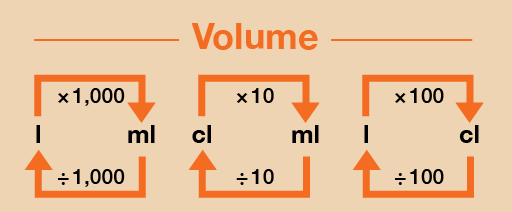Everyday maths 1 (Wales)

Start this free course now. Just create an account and sign in. Enrol and complete the course for a free statement of participation or digital badge if available.

# 4.2 Converting metric units of capacity

You will sometimes need to change between millilitres and litres. There are 1 000 millilitres in a litre.

Take a look at this metric conversion chart to refer to when you are carrying out the activity below.Figure 33 A conversion chart for volume

As mentioned earlier, capacity/volume can be measured in centilitres (cl), but it is more common to use millilitres (ml) and litres (l), so we will focus on converting between these here.

## Example: Converting units of capacity

1. Convert the following from litres into millilitres:
• a.7 litres = ? ml
• b.8.5 litres = ? ml
2. Convert the following from millilitres into litres:
• a.6 000 ml = ? litres
• b.2 750 ml = ? litres

### Method

1. As you can see from Figure 33, to convert from litres (l) to millilitres (ml), you need to multiply by 1 000:
• a.7 l × 1 000 = 7 000 ml
• b.8.5 l × 1 000 = 8 500 ml
2. If you want to convert from millilitres (ml) to litres (l) then you need to divide by 1 000:
• a.6 000 ml ÷ 1 000 = 6 l
• b.2 750 ml ÷ 1 000 = 2.75 l

Now try the following activity.

## Activity 16: Converting metric units of capacity

Calculate the following without using a calculator. You may wish to look back at Session 1 to remind you how to multiply [Tip: hold Ctrl and click a link to open it in a new tab. (Hide tip)] and divide by 1 000. Remember to check your answers.

1. What are the following measurements in litres?
• a.4 000 ml
• b.3 500 ml
• c.650 ml
• d.8 575 ml
2. What are the following measurements in millilitres?
• a.9 litres
• b.2.5 litres
• c.4.8 litres
• d.8.95 litres

1. The answers are as follows:
• a.4 000 ml ÷ 1 000 = 4 litres
• b.3 500 ml ÷ 1 000 = 3.5 litres
• c.650 ml ÷ 1 000 = 0.65 litres
• d.8 575 ml ÷ 1 000 = 8.575 litres
2. The answers are as follows:
• a.9 litres × 1 000 = 9 000 ml
• b.2.5 litres × 1 000 = 2 500 ml
• c.4.8 litres × 1 000 = 4 800 ml
• d.8.95 litres × 1 000 = 8 950 ml#### Live in Wales? Free your ambition with a paid part-time course with the OU in Wales.

With grants of up to £4,500* to help with living costs, and tuition fee loans to cover course fees,
now's the time to take that next step.

#### Find out more

*Eligibility rules apply for financial support.